Figure 6.3 The structure of a gtree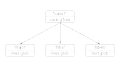R code
 Figure 6.5 Using a gtree to group grobs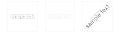R code
 Figure 6.6 Packing grobs by hand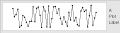R code
 Figure 6.7 Calculating the size of a grob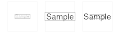R code
 Figure 6.8 Grob dimensions by reference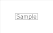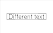R code
 Figure 6.10 Editing the grobs in a lattice plot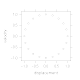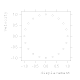R code